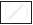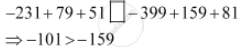Share

# Use the Sign of >, < Or = in the Box to Make the Statements True.– 231 + 79 + 51 and –399 + 159 + 81 - CBSE Class 7 - Mathematics

ConceptIntroduction of Integer

#### Question

Use the sign of >, < or = in the box to make the statements true.

– 231 + 79 + 51–399 + 159 + 81

#### SolutionIs there an error in this question or solution?

#### APPEARS IN

NCERT Solution for Mathematics for Class 7 (2018 to Current)
Chapter 1: Integers
Ex. 1.10 | Q: 9.5 | Page no. 5
Solution Use the Sign of >, < Or = in the Box to Make the Statements True.– 231 + 79 + 51 and –399 + 159 + 81 Concept: Introduction of Integer.
S